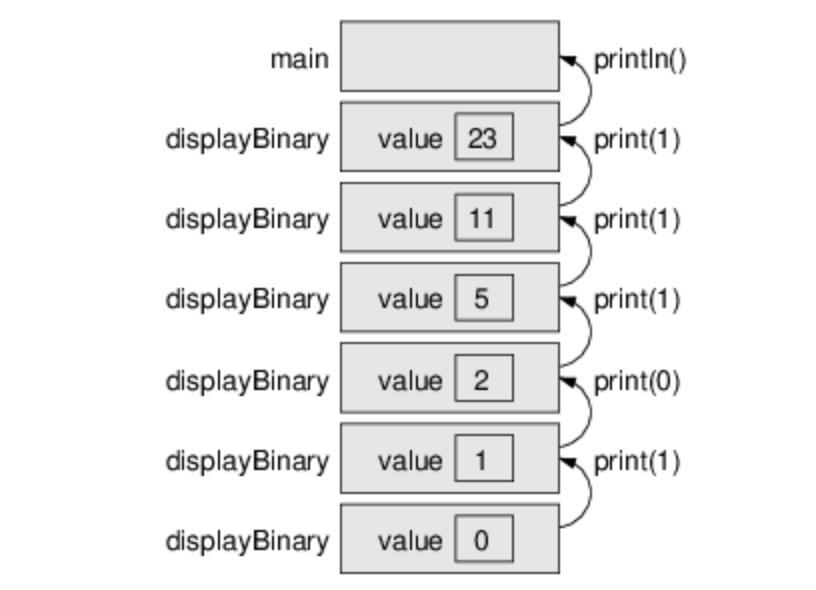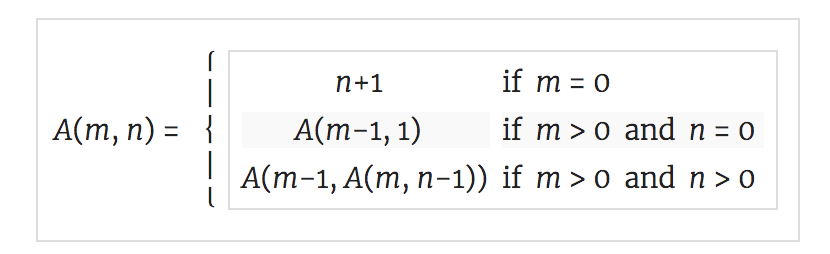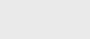10个月前 (10-05) |   抢沙发  147

## 二进制数系统

 Binary Decimal 0 0 1 1 10 2 11 3 100 4 101 5 110 6 111 7

 4 5 6 102 101 100

 1 0 1 1 1 24 23 22 21 20

``````23 / 2 is 11 remainder 1
11 / 2 is  5 remainder 1
5 / 2 is  2 remainder 1
2 / 2 is  1 remainder 0
1 / 2 is  0 remainder 1
``````

## 递归二进制法

``````public static void displayBinary(int value) {
if (value > 0) {
displayBinary(value / 2);
System.out.print(value % 2);
}
}````````````displayBinary(23);      // output is 10111
System.out.println();``````

## CodingBat问题

### 递归-1 noX

``````noX("xaxb") → "ab"

noX("abc") → "abc"

noX("xx") → ""``````

``````if (str.length() == 0) {
return "";
}``````

``````char first = str.charAt(0);
String rest = str.substring(1);``````

``String recurse = noX(rest);``

``````if (first == 'x') {
return recurse;
} else {
return first + recurse;
}``````

### 递归-1数组11

``````array11([1, 2, 11], 0) → 1
array11([11, 11], 0) → 2
array11([1, 2, 3, 4], 0) → 0``````

``````if (index >= nums.length) {
return 0;
}``````

``````int recurse = array11(nums, index + 1);
if (nums[index] == 11) {
return recurse + 1;
} else {
return recurse;
}``````

## 递归练习题

### 使用堆栈图来理解以下递归方法的执行：

``````public static void main(String[] args) {
System.out.println(prod(1, 4));
}

public static int prod(int m, int n) {
if (m == n) {
return n;
} else {
int recurse = prod(m, n - 1);
int result = n * recurse;
return result;
}
}``````
1. 绘制一个堆栈图，显示最后一次`prod`调用完成之前程序的状态。
2. 这个程序的输出是什么？（请先在纸上回答这个问题；然后运行代码检查您的答案。）
3. 用几句话解释一下`prod`是做什么的（没有详细说明它是如何工作的）。
4. 重写`prod`而不使用递归和结果临时变量。提示：`else`分支只需要一行。### 创建一个名为递归.java并键入以下方法：

``````/**
* Returns the first character of the given String.
*/
public static char first(String s) {
return s.charAt(0);
}``````
``````/**
* Returns all but the first letter of the given String.
*/
public static String rest(String s) {
return s.substring(1);
}``````
``````/**
* Returns all but the first and last letter of the String.
*/
public static String middle(String s) {
return s.substring(1, s.length() - 1);
}``````
``````/**
* Returns the length of the given String.
*/
public static int length(String s) {
return s.length();
}``````
1. `main`中编写一些测试这些方法的代码。确保它们能起作用，你也能理解它们的作用。
2. 使用这些方法，在不使用任何其他字符串方法的情况下，编写一个名为`printString`的方法，该方法以字符串为参数并显示字符串的字母，每行一个。它应该是一个无效的方法。
3. 同样，只使用这些方法，编写一个名为`printback`的方法，它执行与`printString`相同的操作，但是向后显示字符串（同样，每行一个字符）。
4. 现在编写一个名为`reverseString`的方法，它将字符串作为参数，并返回一个新字符串作为返回值。新字符串应包含与参数相同的字母，但顺序相反：
``````String backwards = reverseString("coffee");
System.out.println(backwards);``````

``eeffoc``### 注册